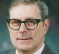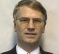## IQ mag: Integer approximation of square root of the sum of two squaresStarted by 11 months ago23 replieslatest reply 10 months ago254 views

Anyone knows the name of this algorithm and a fast and also integer alternative?

Int16 Mag(int16 i, int16 q)

(

If abs(i) > abs(q)

Return abs(i) + abs(q) / 4;

Else

Return abs(q) + abs(i) / 4;

)

[ - ]This is the "Alpha Max + Beta Min" algorithm as a high-speed approximation for the magnitude of a complex number (or more generically the square root of the sum of two squares. Please see https://en.wikipedia.org/wiki/Alpha_max_plus_beta_...

[ - ]Hi Dan. I learned Robmar's algorithm back in the 1980s and was told it was called "Max plus beta min." Back then I thought, with an additional multiply perhaps we could improve that algorithm's performance. So I started experimenting with multiplying the max value by an alpha value where 0 ≤ alpha ≤ 1. I decided to call the modified algorithm "Alpha max plus beta min."

[ - ]Interesting, even more so if you would share your work :)  Thanks!

[ - ]Hello Robmar. My ol' DSP pal Grant Griffin posted material on the Alpha Max Plus Beta Min algorithm at:

[ - ]Very nice Rick! I didn't know that, thanks for the additional history and nice work. Until I reread the post, I was going to go forward from this point thinking that it was originally "Robmar's Algorithm" up until the day you improved it. But now I get that you meant the algorithm that the poster Robmar here described.

[ - ]Thanks for that, very useful!!

[ - ]Dan,

I can't believe you didn't mention these:

Best Approach to Rank Complex Magnitude Comparision Problem

[ - ]Related but those don’t really fit the bill as they are used to compare two complex magnitudes rather than estimate the complex magnitude. However what I didn’t mention but is also an efficient estimator is the CORDIC Rotator. (Use the CORDIC to rotate the angle to zero with the result on I as the magnitude times the CORDIC gain

[ - ]IIRC, some of the methods did this by computing the two values and comparing them.  Anyway, the post covered the OP's equation to some detail as well as many other issues having to do with an integer implementation.

Close enough to be very useful, IMO.  The big challenge there was avoiding any multiplications, hence a lot of shifts in the solutions.

Here is how I think I would do this one:

=====================
prep()

for M = 0 to 2^5
A = atan( M / (2^5) )

XF[M] = 2^7 * cos( A )
YF[M] = 2^7 * sin( A )
next

=====================
mag( I, Q )

AI = abs( I )
AQ = abs( Q )

if AI + AQ = 0 return 0

if AI < AQ then
X = AQ : Y = AI
else
X = AI : Y = AQ

M = ( Y << 5 ) / X

D = ( X * XF[M] + Y * YF[M] ) >> 7

return D

=====================


Here the '5' represents a 2^5 + 1 = 33 place lookup table.

The '7' represents a fixed point fraction offset of 7 in the table values.

Why it works.

---------------
X = R cos( A )
Y = R sin( A )

X * XF + Y * YF = R cos( A ) * cos( A ) + R sin( A ) * sin( A )
= R ( cos^2( A ) + sin^2( A ) )
= R
---------------


You can also think of it as a local plane approximation to sqrt( x^2 + y^2 ), or a rotation of the point to the real axis.

The OP didn't specify any tolerance, so I have no recommendation for the table size.
[ - ]I have known about this for decades, but never saw it called an integer approximation. In the early days of my career, a square root operation was a big expense, but I noticed that most of the time, one ended up comparing the computed square root of the sum of squares to some threshold, and it was easier to compare the sum of the squares to the square of the threshold.

[ - ]It is also worth reflecting on how this affects investigations of signals in noise and the apparent sharpness of the transition.

In a broad band signal you can add 50% noise for only a 10% increase in the total power, and like wise if the signal is a full 50% of the noise, you won't see it's 10% overall contribution.

It you are ever testing for susceptibility you should try 'synchronising' the noise injection to a visible alias frequency of the equipment operating frequency. Unfortunately its not mentioned in any of the standards. Essentially you look to make the injection noise look like 'Hum Bars' on the signal (Hum bars were a feature of US TV sets where the AC supply frequency (and it's harmonic) was a fraction different (apparently by design) from the TV line frequency. Works wherever you have a synchronised 'image' display - because everyone can see the obvious moving injceted noise!

[ - ]I haven't got my head around the varied use of noise injection yet.  Was reading on that in the technique to increases ADC resolution if there was enough noise on the sampled signal, or adding noise, so that a 1 or more but increase could be achieved.  I mean if the signal is so stable that you need noise to flip the LS bit, what's the gain?!  Thanks for your reply

[ - ]Years ago (on a Sinclair QL IIRC) I did calcs regarding adding rms noise equal to 1/2 lsb and found that with sufficient averaging, you got at least 8 extra bits of linearity. You need 2^(2N) samples to get N bits of extra linearity.

I suspect it's also a part of the reason we get DAC / ADC non-linearity limits of a 2-lsb step, so 1 lsb rms (Gaussian) would hide it ;-).

The spike noise injection (e.g. close capacitive coupling) is useful with systems that have sampled ADCs such as cameras and imagers so the aperture time for a single noise spike is low, but if you make it synchronised to a scrolling pattern, the eye will pick it out really easy. Had that happen many years ago with a perfect 600Hz drive signal interfering with an NTSC timed imager system and generating beautiful scrolling bands, even though all the RFI tests passed.

[ - ]There are variations on the integer IQ magnitude approximation (still with compute-friendly coefficient values) that give a lower error that that posted above and also with an error that is better centred about zero. If I recall correctly:

mag ~= 7/8 abs(largest) + 1/2 abs(smallest)

and better still mag ~= 15/16 abs(largest) + 1/2 abs(smallest)

(7/8 and 15/16 can be computed using a shift and a subtract)

On a side note, if you need log magnitude and can afford the time needed for a log10 call then using 10 * log10 (sum of squares) gives you the square root for free.

Tim.

[ - ]I learned something today!  Updated my spreadsheet to confirm :)

 I Q SOS Z =sqrt((I*I)+(Q*Q)) 20*log10(Z) 10*log10(SOS) “Mag” function % error 20 0.01 400.000 20.000 26.021 26.021 20.0025 0.012 20 1 401.000 20.025 26.031 26.031 20.25 1.124 20 5 425.000 20.616 26.284 26.284 21.25 3.078
Robert
[ - ]Thanks Tim, that's helpful, I'll give those a try!

[ - ]Check out this post:

Same math, different reason.

[ - ]Thanks for the link, its an interesting post, but too many CPU cycles!

[ - ]I guess with z = sqrt( ( I * I ) + ( Q * Q ) ), as Q becomes <<< I, the contribution from Q becomes less and less, and the result approaches sqrt( I * I ), or abs(I), with some change, which is the abs(Q)/4.  And vice versa.

I did some quick spreadsheetin' as empirical proof:

 I Q z Mag fn % error 20 0.01 20.000 20.0025 0.012 20 1 20.025 20.25 1.124 20 5 20.616 21.25 3.078

I think this is already a fast approximation to the full sqrt operation (sqrt being costly in CPU cycles), using integer values.  The big hit in your routine is in the pipeline-clearing logical If-Else.  If you knew for certain that Q was always <<< I, or vice versa, you could get rid of that by just using one of those two Return statements.

[ - ][ - ]Here is an improved answer over the one in my earlier reply to Dan.

The '7' is actually the number of effective significant bits.  Headroom calculations are application dependent.

Otherwise, without too large a table, you should be able to get very reasonable results.

=====================
prep()

for M = 0 to 2^5
A = atan( M / (2^5) )

Cos_M[M] = 2^7 * cos( A )
Sin_M[M] = 2^7 * sin( A )
next

=====================
mag( I, Q )

AI = abs( I )
AQ = abs( Q )

if AI < AQ then
M = ( AI << 5 ) / AQ
D = ( AQ * Cos_M[M] + AI * Sin_M[M] ) >> 7
else
if AI = 0 return 0
M = ( AQ << 5 ) / AI
D = ( AI * Cos_M[M] + AQ * Sin_M[M] ) >> 7

return D

=====================

[ - ]This answer is what you might be looking for.

https://dsp.stackexchange.com/questions/62893/#630...

It divides the arc up without the division, and multiplies the coefficients with hard coded shifts and adds.

Since you are "counting cycles", I would say, "test it, and time it" instead.

[ - ]In your code, abs() is called six times when you need to do it just twice and also MUL usually is cheaper than DIV so /4 could be changed to *0.25. How much you could gain in execution speed depends on your compiler/-options... . If you do it using C++, current GCC and Clang compilers also does good job with those std library functions (maybe with sqrt() and abs() too (I have not bench-marked these two)).

Looks like (when compiled using C++/GCC -O3 -ffast-math) library sqrt() as

int16_t intsqrt(int16_t x, int16_t y){
return sqrt(x * x + y * y);
}

is just little (~0.3 rdtsc/val) slower than function (with little modifications mentioned in discussion) given in opening post, so, why approximate (of course if you use other programming language or compiler which does not do good optimization...)?

[ - ]